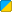# table.Count

 Function Syntax table.Count( Table ) Description: Gets the total number of keys in a table - including both integer-keys and string-keys. Returns: Integer The number of keys in the table. Part of Library: Table Realm:BBCode Link: [b][url=http://wiki.garrysmod.com/?title=Table.Count]Table.Count [img]http://wiki.garrysmod.com/favicon.ico[/img][/url][/b]

## Example

Description Prints a few various conditions involving table.Count and a table.```  test_table = {"this","that","and the other"} // Define the table. print(table.Count(test_table)) // Prints "3" to the console, how many keys are within test_table. table.insert(test_table,"hello") // Inserts a new value. print(table.Count(test_table)) // Last addition is now 4. test_table = "test" test_table[-1] = "Negative number." print(table.Count(test_table)) // Prints 6, 0 and -1 are counted. test_table["hello"] = "test" print(table.Count(test_table)) // Prints 7.  ``` Four numbers to the console of different situations in the script.

• This function loops through the entire table every time it is run - be warned this can possibly cause infinite loop protection to kick in on large tables, and is overall inefficient for use in large tables. It is suggested you use table.getn if your table is integer indexed.
• This function does not check to see if the table is nil, and so will return an error if it is.
• If the table is indexed with consecutive integers use the length operator instead. (#table)